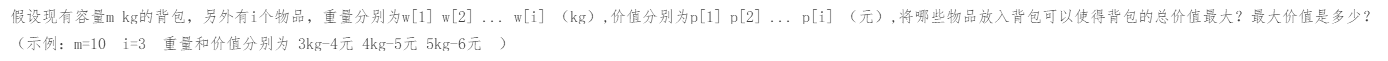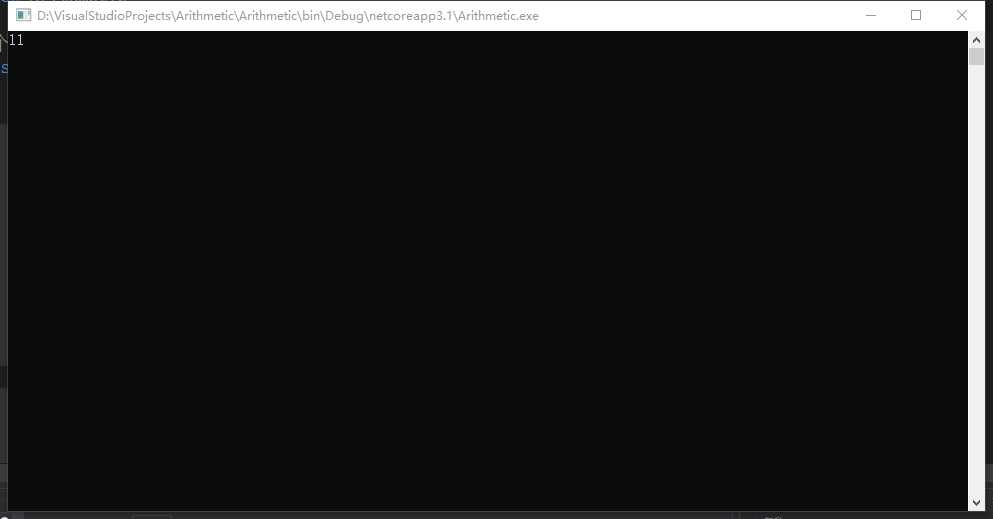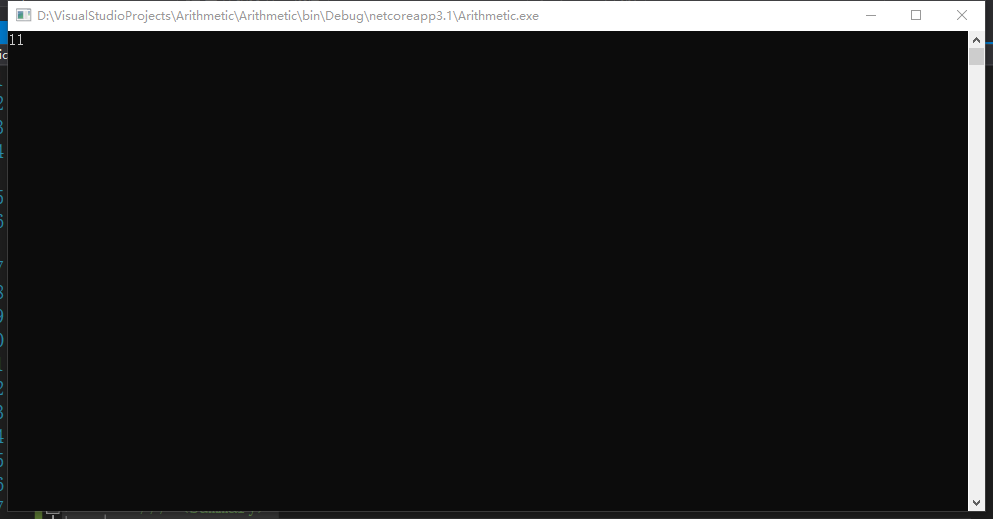### 01背包问题理解动态规划算法1.穷举法

```        static void Main(string[] args)
{
//记录重量的数组，物品有3种重量，分别是3、4、5
int[] w = { 0, 3, 4, 5 };
//记录物品价值的数组，和重量数组对应，物品价值分别是4、5、6
int[] p = { 0, 4, 5, 6 };
//调用Exhaustivity函数得到7kg的背包放置物品的最大价值
Console.WriteLine(Exhaustivity(9 , w, p));
Console.ReadKey();
}
/// <summary>
/// 计算给定的mkg背包放置物品的最大价值
/// </summary>
/// <param name="m">给定的物品重量</param>
/// <param name="w">记录所有物品重量的数组</param>
/// <param name="p">记录所有物品对应价格的数组</param>
/// <returns>背包中放置物品的最大价值</returns>
public static int Exhaustivity(int m,int[] w,int[] p)
{
//记录放入物品的最大的价格
int maxPrice = 0;
//有3个物品，外层就循环2的3次方，即i值从0取到7，i的二进制数字最多3位，每一个i的取值的二进制数字都对应3个物品是否放入背包
//如i为6，二进制为110，代表取前两个物品，不取第3个物品
//又如i为0，二进制为000，代表3个物品均不取；i为1，二进制001，代表只取第3个物品
//通过这种方式穷举所有物品放入背包的情况，然后判断每一种情况重量是否满足要求，再比较价格
for(int i = 0;i < Math.Pow(2,w.Length - 1); i++)
{
//记录所有被放入背包物品的总重量
int weightTotal = 0;
//记录所有被放入背包物品的总价格
int priceTotal = 0;
//内层循环负责计算并比较所有物品的总重量和总价格
//内层循环的次数就是物品个数，然后分别判断当前情况下每个物品是否放入背包，j代表第几个物品
for(int j = 1;j <= w.Length - 1; j++)
{
//计算当前物品是否放入背包
int result = Get2(i, j);
//在放入背包的情况下，将重量和价格累加到总重量和总价格上
if(result == 1)
{
weightTotal += w[j];
priceTotal += p[j];
}
}
//判断是否超重，不超重的情况下判断价格是不是大于最大价格，如果是更新最大价格
if (weightTotal <= m && priceTotal > maxPrice)
maxPrice = priceTotal;
}
//返回最大价格
return maxPrice;
}
/// <summary>
/// 通过按位与运算得到给定物品number在给定放入情况i中是否放入背包
/// </summary>
/// <param name="i">给定的物品放入情况</param>
/// <param name="number">给定的物品编号</param>
/// <returns></returns>
public static int Get2(int i,int number)
{
int A = i;
int B = (int)Math.Pow(2,number - 1);
int result = A & B;
if (result == 0)
return 0;
return 1;
}```2.分治算法（自上而下分解问题）

这个问题的分治算法的核心是将物品逐个放入背包，有以下几种情况：

1）背包没有剩余重量了

2）物品太重，无法放入背包

3）物品的编号为0，即所有的物品都试过了

4）物品还可以放入背包

```        static void Main(string[] args)
{
//记录重量的数组，物品有3种重量，分别是3、4、5
int[] w = { 0, 3, 4, 5 };
//记录物品价值的数组，和重量数组对应，物品价值分别是4、5、6
int[] p = { 0, 4, 5, 6 };
Console.WriteLine(UpDown(9, w.Length - 1, w, p));
Console.ReadKey();
}
/// <summary>
/// 根据背包剩余重量和物品编号计算放入物品能获得最大价值还是不放入物品能获得最大价值
/// </summary>
/// <param name="m">背包剩余能装的重量</param>
/// <param name="i">当前放入背包的物品编号</param>
/// <param name="w">记录所有物品重量的数组</param>
/// <param name="p">记录所有物品价格的数组</param>
/// <returns>放入背包的物品的最大价格</returns>
public static int UpDown(int m,int i,int[] w,int[] p)
{
//如果背包还能装的重量为0或者放入的物品编号为0，价格自然都是0
if (i == 0 || m == 0) return 0;
//将第i个物品放入背包，但是物品放入后超重，这种情况下就不放入这个物品，看下一个物品是否能放入背包（物品编号-1）
if(w[i] > m)
return UpDown(m,i - 1, w, p);
//在第i个物品能放入背包的情况下需要计算比较价格
else
{
//计算并记录将物品放入背包的情况下的最大价格（递归物品编号-1，从背包剩余重量中扣除当前物品重量，由于当前物品放入背包，背包中物品价格加上当前物品价格）
int maxValue1 = UpDown(m - w[i], i - 1, w, p) + p[i];
//计算并记录不将物品放入背包的情况下的最大价格（递归物品编号-1，但是由于没有放入物品，背包剩余重量不变）
int maxValue2 = UpDown(m, i - 1, w, p);
//比较并取其中价格最大的那个
if (maxValue1 > maxValue2)
return maxValue1;
return maxValue2;
}
}```3.改进分治算法，使用数组记录已经运算过的问题结果

```        //定义一个规则二维数组记录结果，行数对应背包重量，列数对应放入的物品个数，值对应这种情况背包中的最大重量
public static int[,] result = new int[11, 4];
static void Main(string[] args)
{
int[] w = { 0, 3, 4, 5 };
int[] p = { 0, 4, 5, 6 };
Console.WriteLine(UpDown(9, w.Length - 1, w, p));
Console.ReadKey();
}
public static int UpDown(int m,int i,int[] w,int[] p)
{
if (i == 0 || m == 0) return 0;
//判断这种情况是不是已经计算过了，计算过了就直接返回
if (result[m, i] != 0)
return result[m, i];
//在计算完成后需要将计算结果存储起来
if(w[i] > m)
return result[m,i] = UpDown(m,i - 1, w, p);
else
{
int maxValue1 = UpDown(m - w[i], i - 1, w, p) + p[i];
int maxValue2 = UpDown(m, i - 1, w, p);
if (maxValue1 > maxValue2)
return result[m, i] = maxValue1;
return result[m, i] = maxValue2;
}
}```

4.动态规划算法（自下而上计算问题）

```        static void Main(string[] args)
{
int[] w = { 0, 3, 4, 5 };
int[] p = { 0, 4, 5, 6 };
Console.WriteLine(BottomUp(9, w.Length - 1, w, p));
Console.ReadKey();
}
//记录运算结果的数组，行数代表背包重量，列数代表物品编号
public static int[,] result = new int[11, 4];
/// <summary>
/// 计算指定重量m的背包种放置前i个物品放置的最大价值
/// </summary>
/// <param name="m">背包重量</param>
/// <param name="i">物品编号</param>
/// <param name="w">记录所有物品重量的数组</param>
/// <param name="p">记录所有物品价格的数组</param>
/// <returns></returns>
public static int BottomUp(int m,int i,int[] w,int[] p)
{
//外层循环从1到m，背包重量从小到大
for(int tempM = 1;tempM <= m; tempM++)
{
//内层循环从1到i，物品个数从1到i
for(int tempI = 1;tempI <= i;tempI++)
{
//如果已经计算过，不用再计算了
if (result[tempM, tempI] != 0) continue;
//如果当前物品重量超过背包剩余可装重量，不放入物品，将物品编号减一
if (w[tempI] > tempM)
result[tempM, tempI] = result[tempM, tempI - 1];
//当前放置的物品重量不超过背包剩余可装重量，说明物品能放入背包
//物品不一定放入背包最后的总价值最大，所以需要比较放入和不放入的情况得到的价格哪种更大
else
{
int maxValue1 = result[tempM - w[tempI], tempI - 1] + p[tempI];
int maxValue2 = result[tempM, tempI - 1];
result[tempM, tempI] = maxValue1 > maxValue2 ? maxValue1 : maxValue2;
}
}
}
//自下而上计算完成后，返回重量为m的背包放入i个物品的最大价格即可
return result[m, i];
}```